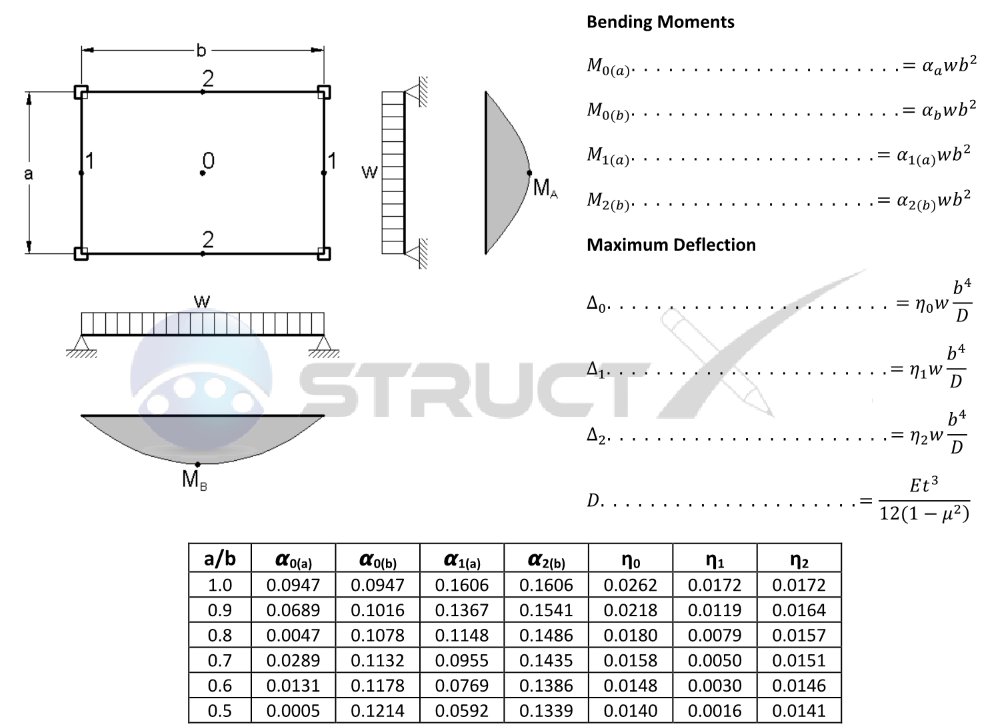# Supported On All Corners With UDLMore Plates# Notation and Units

## Metric and Imperial Units

The above plate formulas may be used with both imperial and metric units. As with all calculations care must be taken to keep consistent units throughout with examples of units which should be adopted listed below. It should be noted that the above formulas and tables are best suited for rectangular plates where b≤2a and that other cases could have better results by considering the plate as a beam instead.

# Notation

• a = shortest span length, in or mm
• b = longest span length, in or mm
• E = modulus of elasticity, psi or MPa
• Mi = maximum bending moment, lbf.in/in or Nmm/mm
• t = plate thickness, mm or in
• w = load per unit area, psi or MPa
• i = deflection or deformation, in or mm
• αi = length to width ratio coefficient
• ηi = length to width ratio coefficient
• μ = poisson's ratio of plate material, assumed to be 1/6 for coefficients